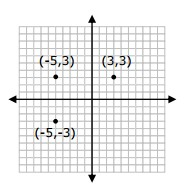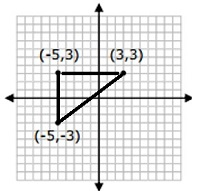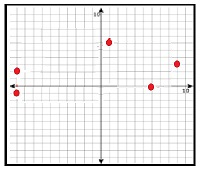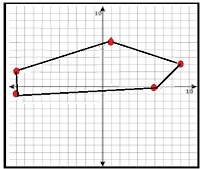# Drawing and identifying a polygon in the coordinate plane

In this lesson we are given the coordinates of points in a coordinate plane.

Joining these points with line segments we get a polygonal figure. We are required to identify the polygon so formed.

Plot the following points (-5, 3) (3,3) (-5,-3) in the coordinate plane. Join the points with line segments and identify the polygonal figure so formed.### Solution

Step 1:

We know that in a coordinate plane, a positive number tells us to move right or up and a negative number tells us to move left or down.

Step 2:

To locate (-5, 3) (3,3) (-5,-3) points

Point (-5, 3): The x coordinate is -5 so we move left 5 units on x axis from origin and since y coordinate 3 is positive, we move up 3 units to locate point (-5, 3):

Similarly, the other two points (3,3) (-5,-3) are located.

Step 3:

Joining the three points with line segments, we see that we get a triangle as shown below.Identify the polygon formed by the points in the coordinate plane below### Solution

Step 1:

There are five points in the given coordinate plane.

Step 2:

Joining these points with line segments, the polygon formed has five sides and is a Pentagon.